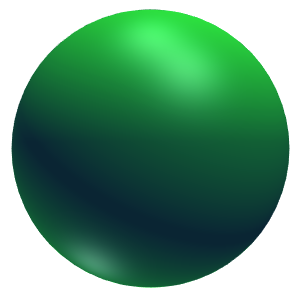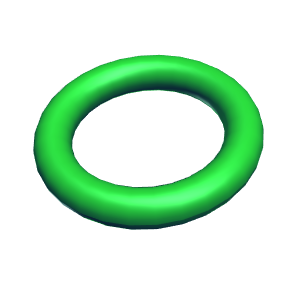### Free Ginckers

#### Revolution: Bullet

Template: Graphics/3d-shape-of-revolution

Discription: Bullet shape created by rotating a plan curve described using a set of parametric equations around a rotation axis that lies on the same plan.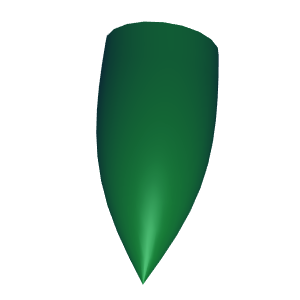#### Revolution: cone

Template: Graphics/3d-shape-of-revolution

Discription: Cone shape created by rotating a plan curve described using a set of parametric equations around a rotation axis that lies on the same plan.#### Revolution: Conical Frustrum

Template: Graphics/3d-shape-of-revolution

Discription: Conical frustrum shape created by rotating a plan curve described using a set of parametric equations around a rotation axis that lies on the same plan.#### Revolution: Cosine

Template: Graphics/3d-shape-of-revolution

Discription: Cosine shape created by rotating a plan curve described using a set of parametric equations around a rotation axis that lies on the same plan.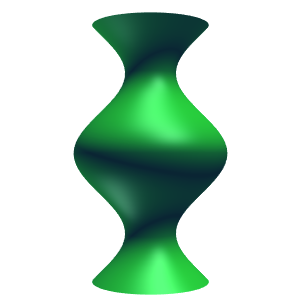#### Revolution: Cosine 2

Template: Graphics/3d-shape-of-revolution

Discription: Another cosine shape created by rotating a plan curve described using a set of parametric equations around a rotation axis that lies on the same plan.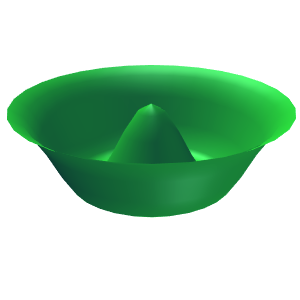#### Revolution: Cylinder

Template: Graphics/3d-shape-of-revolution

Discription: Cylinder shape created by rotating a plan curve described using a set of parametric equations around a rotation axis that lies on the same plan.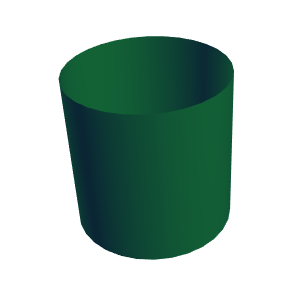#### Revolution: Egg

Template: Graphics/3d-shape-of-revolution

Discription: Egg shape created by rotating a plan curve described using a set of parametric equations around a rotation axis that lies on the same plan.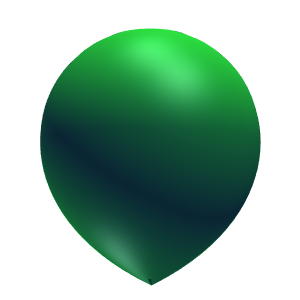#### Revolution: Eight Figure

Template: Graphics/3d-shape-of-revolution

Discription: Eight figure created by rotating a plan curve described using a set of parametric equations around a rotation axis that lies on the same plan.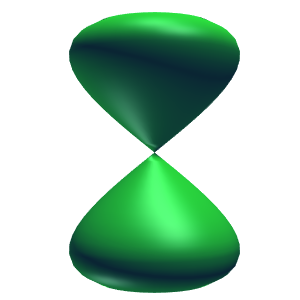#### Revolution: Hyperbolic

Template: Graphics/3d-shape-of-revolution

Discription: Hyperbolic shape created by rotating a plan curve described using a set of parametric equations around a rotation axis that lies on the same plan. Various shapes can be created by changing parameters.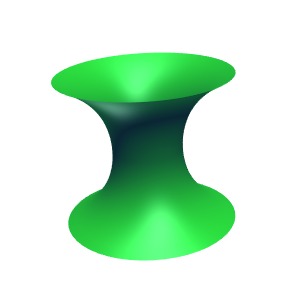#### Revolution: Parabola

Template: Graphics/3d-shape-of-revolution

Discription: Biquadrate parabola created by rotating a plan curve described using a set of parametric equations around a rotation axis that lies on the same plan.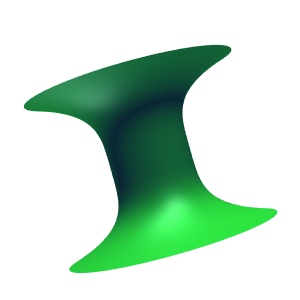#### Revolution: Parabolic Humming-Top

Template: Graphics/3d-shape-of-revolution

Discription: Parabolic Humming-top created by rotating a plan curve described using a set of parametric equations around a rotation axis that lies on the same plan. Various shapes can be created by changing parameters.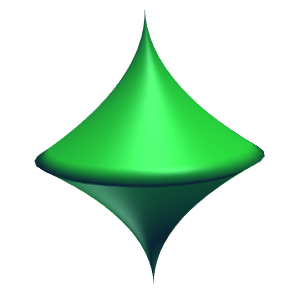#### Revolution: Parabolic Log

Template: Graphics/3d-shape-of-revolution

Discription: Parabolic log created by rotating a plan curve described using a set of parametric equations around a rotation axis that lies on the same plan. Various shapes can be created by changing parameters.#### Revolution: Pear

Template: Graphics/3d-shape-of-revolution

Discription: Pear created by rotating a plan curve described using a set of parametric equations around a rotation axis that lies on the same plan. Various shapes can be created by changing parameters.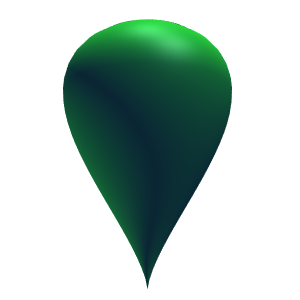#### Revolution: Sine Square

Template: Graphics/3d-shape-of-revolution

Discription: Sine square shape created by rotating a plan curve described using a set of parametric equations around a rotation axis that lies on the same plan. Various shapes can be created by changing parameters.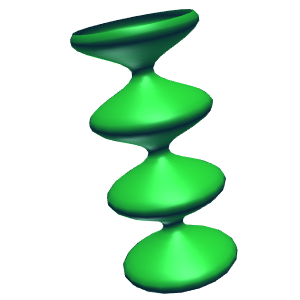#### Revolution: Sphere

Template: Graphics/3d-shape-of-revolution

Discription: Sphere created by rotating a plan curve described using a set of parametric equations around a rotation axis that lies on the same plan. Various shapes can be created by changing parameters.